#ver. 8.1

last update; Aug. 24. 2018

## Calculation of gene coexpression

### Data source

• For human (c3.1); 4401 GeneChip data (GPL570, 123 experiments) downloaded from NCBI GEO.
• For mouse (c2.1); 2226 GeneChip data (GPL1261, 154 experiments) downloaded from NCBI GEO.
• For rat (c2.0); 632 GeneChip data (GPL1355, 22 experiments) downloaded from NCBI GEO.

### Normalization

1. RMA normalization was applied to experiments (123 for human and 154 for mouse).
2. Genes were normalized by those expression levels in each of the experiments.
3. All experiments were combined into one gene expression table for human and mouse, respectively.

### Calculation of sample redundancy

Some data such as a large series of time-course experiments under a single biological condition are biologically redundant or biased. Since these biases may mislead to incorrect conclusions, we have corrected these possible redundancies and biases based on Pearson's correlation coefficients (PCCs) between samples.

1. First, PCCs between sample S1 and sample S2 were calculated.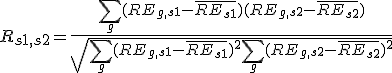, where REg,s is the relative expression of gene G in sample S,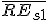is the average relative expression value for all genes in sample S1;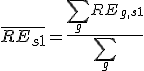,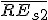is the average relative expression value for all genes in sample S2;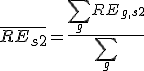.

2. For the paiwise sample redundancy (Js1,s2) between sample S1 and sample S2, we introduced the cut-off threshold C to Rs1,s2.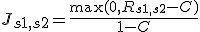.
We used 0.4 for this threshold, which is roughly optimized.

3. The sample redundancy Js1 for sample S1 is calculated as the summation of the pairwise sample redundancies between sample S1 and each of all samples including sample S1 itself.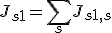4. The weight of sample S1 is the inverse of the square root of the sample redundancy Js1. This procedure is analogous to the calculation of the standard error from the standard deviation. If sample S1 is replicated 4 times with no experimental error, the reliability of the data for sample S1 become double.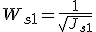### Correlation between probes

The weighted PCC (CORg1,g2) was calculated between probe G1 and probe G2.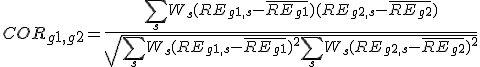, where REg,s is relative expression of probe G in sample S,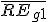is the weighted average relative expression value of probe G1;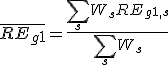,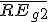is the weighted average relative expression value of probe G2;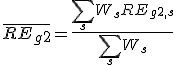.

### Correlation between genes

Maximum correlation value between all probe combination between two genes was used for correlation between two genes.